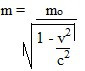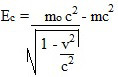Modern Physics

# Relativistic energy

Relativistic energy was described by Einstein in 1905. It provides an expression for the equivalence between mass and energy of a body.

Relativistic energy was discovered by Einstein in 1905. This theory shows that mass and energy are equivalent quantities, and any mass has energy associated with it and vice versa. Mathematically, this relationship is defined by the famous Einstein equation:

E = mc 2

Being:

E – energy of a particle;
m – particle mass;
c – speed of light in vacuum.

The above equation is the best known in Physics and had enormous consequences, among which we can highlight nuclear energy.

Although this energy is bound to a velocity (c), it also provides the rest energy E 0 of a body in a frame of reference by virtue of its rest mass m 0.

0 = m 0. c 2

However, if the body is in motion, with a speed v, we must take into account that it also has a kinetic energy E c and its relativistic mass undergoes expansion, increasing an amount Δm = m – m 0 .

The relativistic mass is given by the equation:The kinetic energy being given by the expression:

And C = Δm . c 2
= ( m – m ) . c 2
= mc – m 2

Don’t stop now… There’s more after the publicity 😉

Substituting m for the relativistic mass expression, we get an expression for the relativistic kinetic energy of this body:This expression shows that a body has energy even if it is at rest just because it has mass, and the factor c 2 can be understood as a conversion factor between the units of the two quantities.

The consequences of relativistic energy

The relativistic energy equation shows that the maximum energy a body can possess is obtained by multiplying mass by the speed of light squared. Which also means that a tiny amount of mass can produce immense amounts of energy. For example: A body with mass m=1.0 kg can produce 9.0 kg. 10 16 J of energy.

This concept of equivalence between mass and energy was used in the development of the atomic bomb, as it allows the calculation of the energy that can be released in a nuclear reaction. This discovery was instrumental in the creation of the two atomic bombs that destroyed the cities of Hiroshima and Nagasaki and that led Einstein to be known as the father of the atomic bomb.